PSAT Math : Arithmetic

Example Questions

Example Question #53 : Proportion / Ratio / Rate

Marty drove 40 mi/hr for 3 hours, then 60 mi/hr for 1 hour, and finally 70 mi/hr for the last 2 hours. What was Marty's average speed?

63 mi/hr

60 mi/hr

53 mi/hr

47 mi/hr

58 mi/hr

53 mi/hr

Explanation:

Marty's total driving time was 3 + 1 + 2 = 6 hours. He drove 40 mi/hr for 3 hours, or 3/6 = 1/2 of the time. He drove 60 mi/hr for 1 hour, or 1/6 of the drive. Lastly, he drove 70 mi/hr for 2 hours, or 2/6 = 1/3 of the drive.

To find the average speed, we need to multiply the speeds with their corresponding weights and add them up.

Average = 1/2 * 40 + 1/6 * 60 + 1/3 * 70 = 53.33... ≈ 53 mi/hr

Example Question #1 : How To Find The Ratio Of A Fraction

A deck of cards has 52 cards. What is the probability of dealing a card with a face value of 4?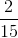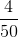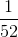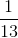Explanation:

For this problem we need to recall that that there are 4 different suits in a deck of cards. Each suit has numbers 1 through 10, jack, queen, king, and ace. Therefore, we can write a ratio of number of 4's over total cards in the deck.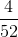From here we can reduce the fraction and we getExample Question #2 : How To Find The Ratio Of A Fraction

There is a 5 to 3 ratio of red marbles to green marbles in a bucket. How many red marbles can be in a bucket of 40 marbles?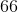Explanation:

In this problem, we use a proportion to solve for the number of red marbles in the bucket. Since the ratio is 5 to 3 we can set up a proportion of the red over total where the total is (red marbles  + green marbles). We then set this proportion equal toover our new total, which is 40. Then we cross multiply and divide to get the number of red marbles in a bucket of 40 total marbles.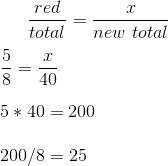Example Question #3 : How To Find The Ratio Of A Fraction

There is a 2 to 3 ratio of dogs to cats in a park. How many cats are there if there are 12 dogs?Explanation:

We set up the following ratio of dogs to cats and set it equal to the new ratio we are interested in.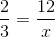Then we cross multiply and divide to solve for.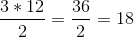Example Question #4 : How To Find The Ratio Of A Fraction

You have a bag filled with red and green apples. If there are 9 red apples and 12 green apples, what is the probability of choosing a red apple out of the bag?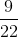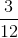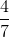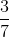Explanation:

To find the probability we need to set up a ratio. The ratio will be how many red apples are in the bag over the total number of apples (both red and green) in the bag.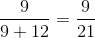We can now reduce this fraction: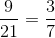Example Question #3 : How To Find The Ratio Of A Fraction

A pie is made up ofcrust,apples, andsugar, and the rest is jelly. What is the ratio of crust to jelly?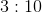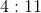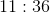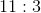Explanation:

A pie is made up ofcrust,apples,sugar, and the rest is jelly. What is the ratio of crust to jelly?

To compute this ratio, you must first ascertain how much of the pie is jelly. This is: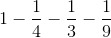Begin by using the common denominator: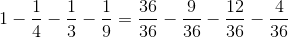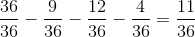So, the ratio of crust to jelly is: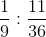This can be written as the fraction: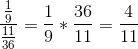, orExample Question #4 : How To Find The Ratio Of A Fraction

In a solution,of the fluid is water,is wine, and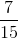is lemon juice. What is the ratio of lemon juice to water?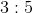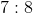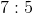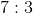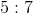Explanation:

This problem is really an easy fraction division. You should first divide the lemon juice amount by the water amount: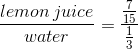Remember, to divide fractions, you multiply by the reciprocal: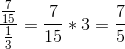This is the same as saying:Example Question #5 : How To Find The Ratio Of A Fraction

If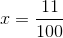and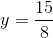, what is the ratio ofto?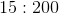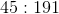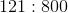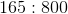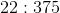Explanation:

To find a ratio like this, you simply need to make the fraction that represents the division of the two values by each other. Therefore, we have: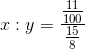Recall that division of fractions requires you to multiply by the reciprocal: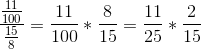which is the same as: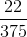This is the same as the ratio:Example Question #1 : How To Find Proportion

The price of 10 yards of fabric is c cents, and each yard makes q quilts. In terms of q and c, what is the cost, in cents, of the fabric required to make 1 quilt?

(cq )/(10 )

10cq

(c )/(10q )

(10c )/(q )

(c )/(10q )

Explanation:

We create a conversion ratio that causes yards to cancel out, leaving only cents in the numerator and quilts in the denominator. This ratio is ((c cent )/(10 yard))((1 yard)/(q quilt))=(c )/(10q ) cent⁄quilt . Since the ratio has cents in the numerator and quilts in the denominator, it represents the price in cents per quilt.

Example Question #2 : How To Find Proportion

Susan is doing a bake sale for her sorority. One third of the money she made is from blueberry cupcakes, which cost 50 cents each. A quarter of her sales is from cinnamon cream pies, which cost $1 each. And the rest are from her chocolate brownies, which cost 25 cents each. She made a total of$60 at the end of her bake sale, how many brownies did she sell?

100

120

130

150

140

1/3 of sales from cupcakes = $20, ¼ of sales from cream pies =$15 and the rest are from brownies = $60-$20-$15 =$25. Since each brownie costs 25 cents, Susan will have sold 100 of them.